# RS Aggarwal Solutions for Class 8 Maths Chapter 1 - Rational Numbers Exercise 1D

Refer and download RS Aggarwal Solutions for Class 8 Maths Chapter 1- Exercise 1D, Rational Numbers from the links provided below. BYJU’S expert team have solved the RS Aggarwal Solutions in order to help students in understanding the concepts and by practicing the solutions, which would help them in scoring good marks in their exams.

In Exercise 1D of RS Aggarwal Class 8 Maths, we shall study the Multiplication of Rational Numbers and properties of Multiplication of Rational Numbers.

In properties, we see Closure property, Commutative Law, Associative Law, Existence of Multiplicative Identity, Existence of Multiplicative Inverse, Distribution Law of Multiplicative Over Addition, Multiplicative Property of 0.

## Download PDF of RS Aggarwal Solutions for Class 8 Maths Chapter 1- Rational Numbers Exercise 1D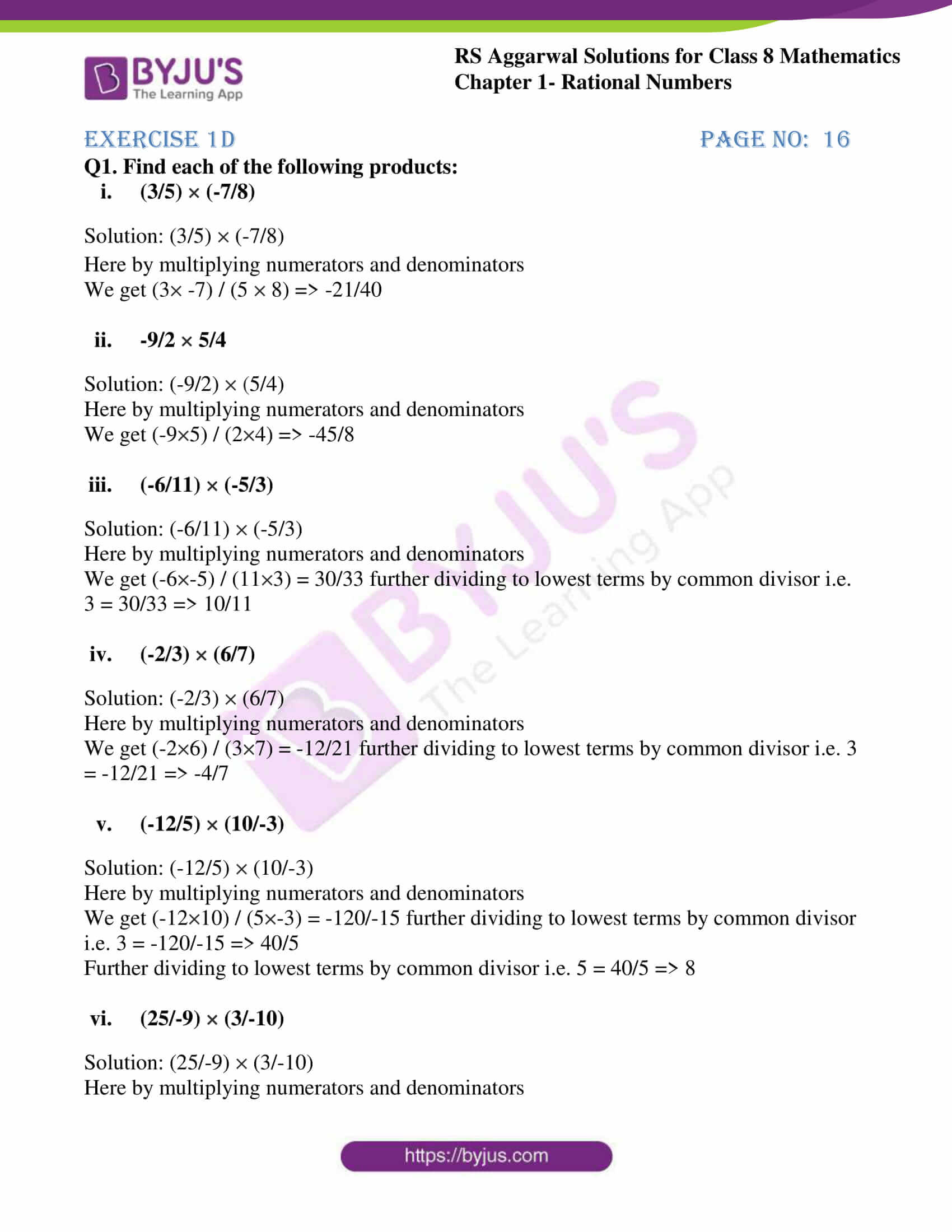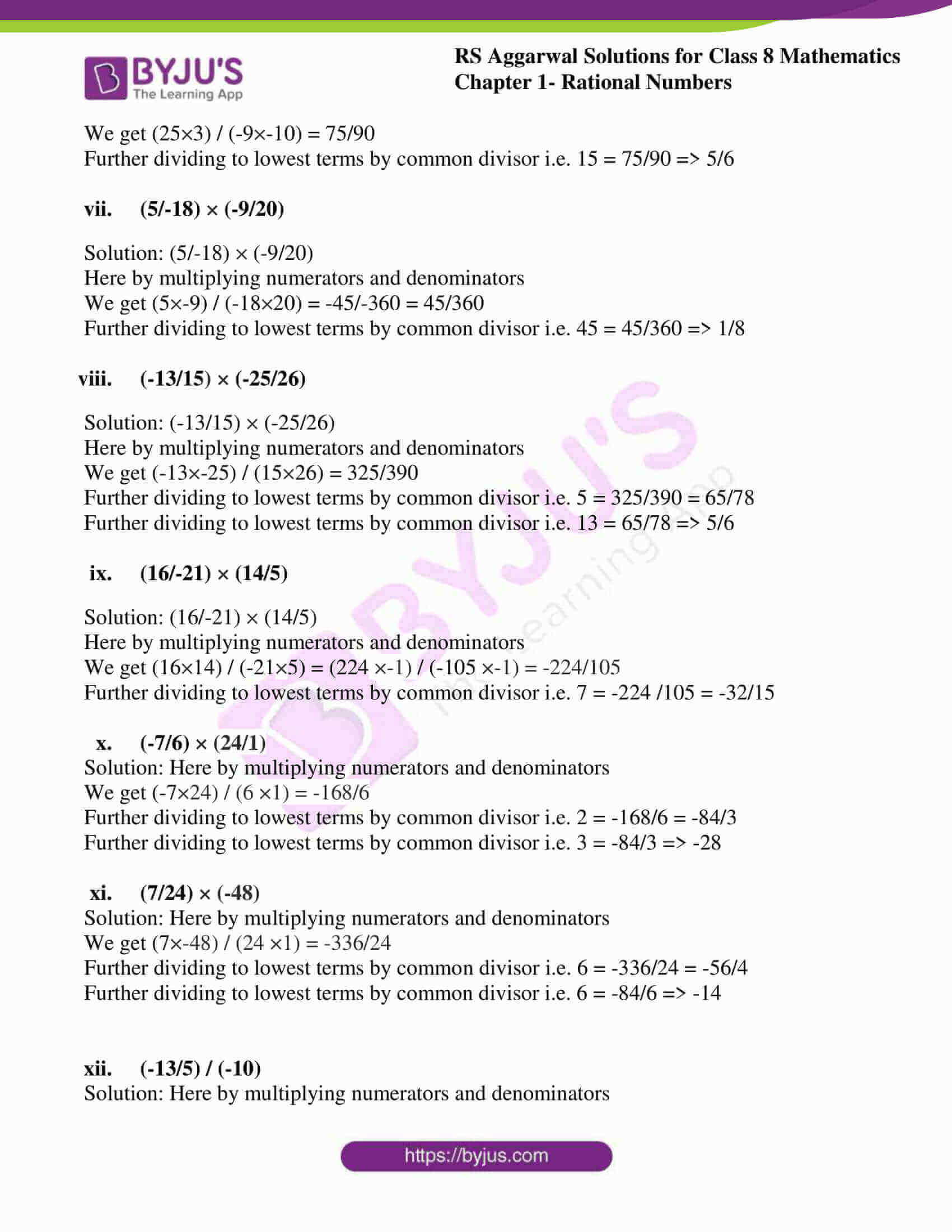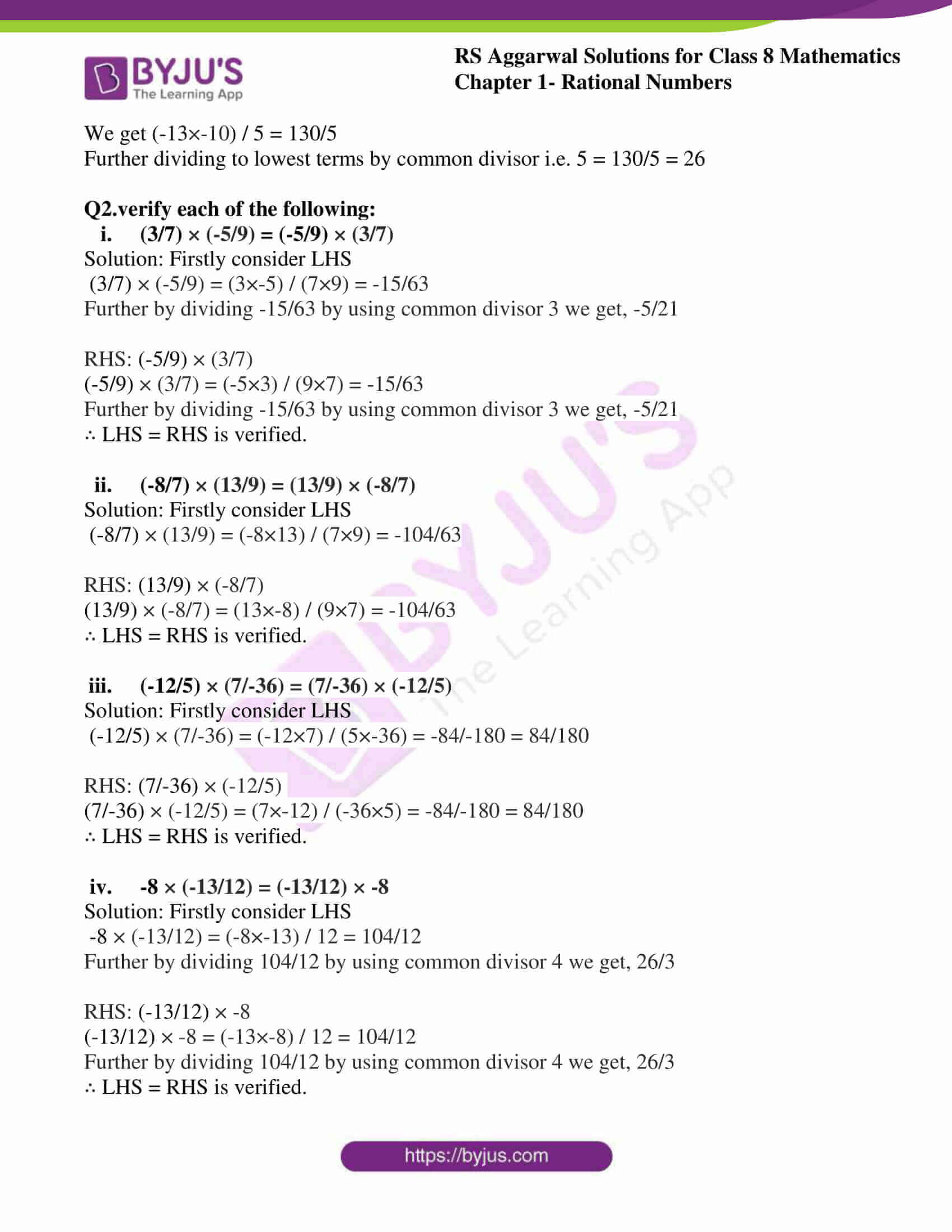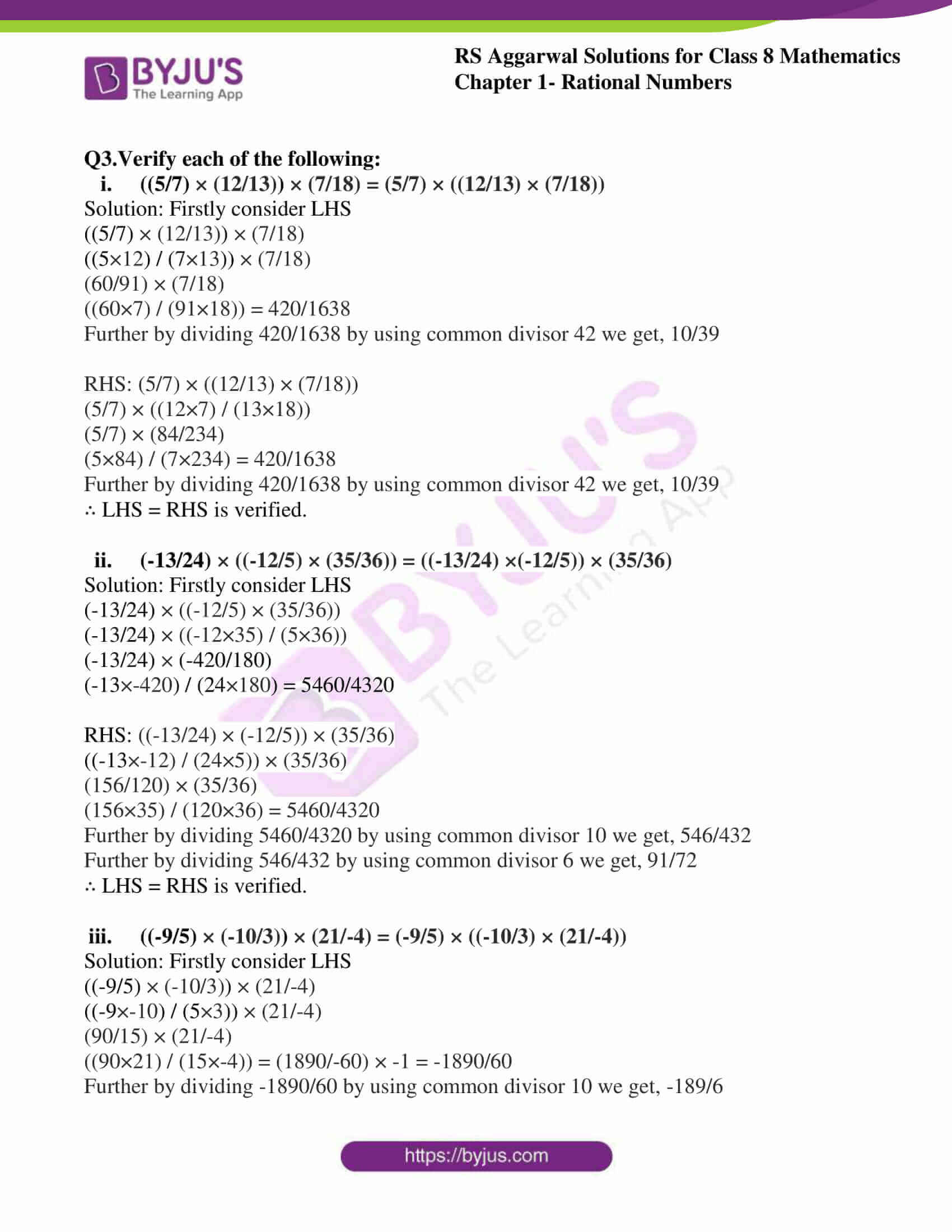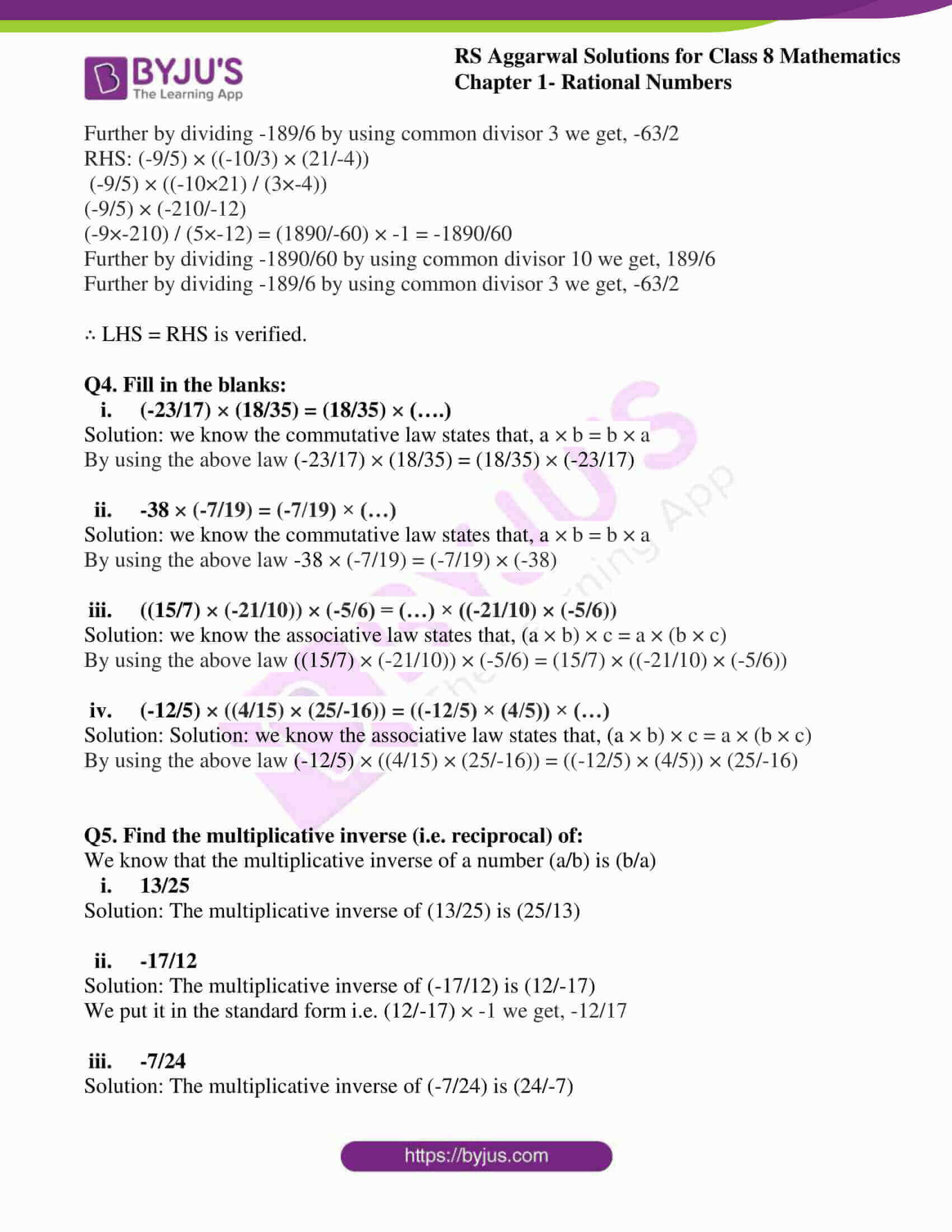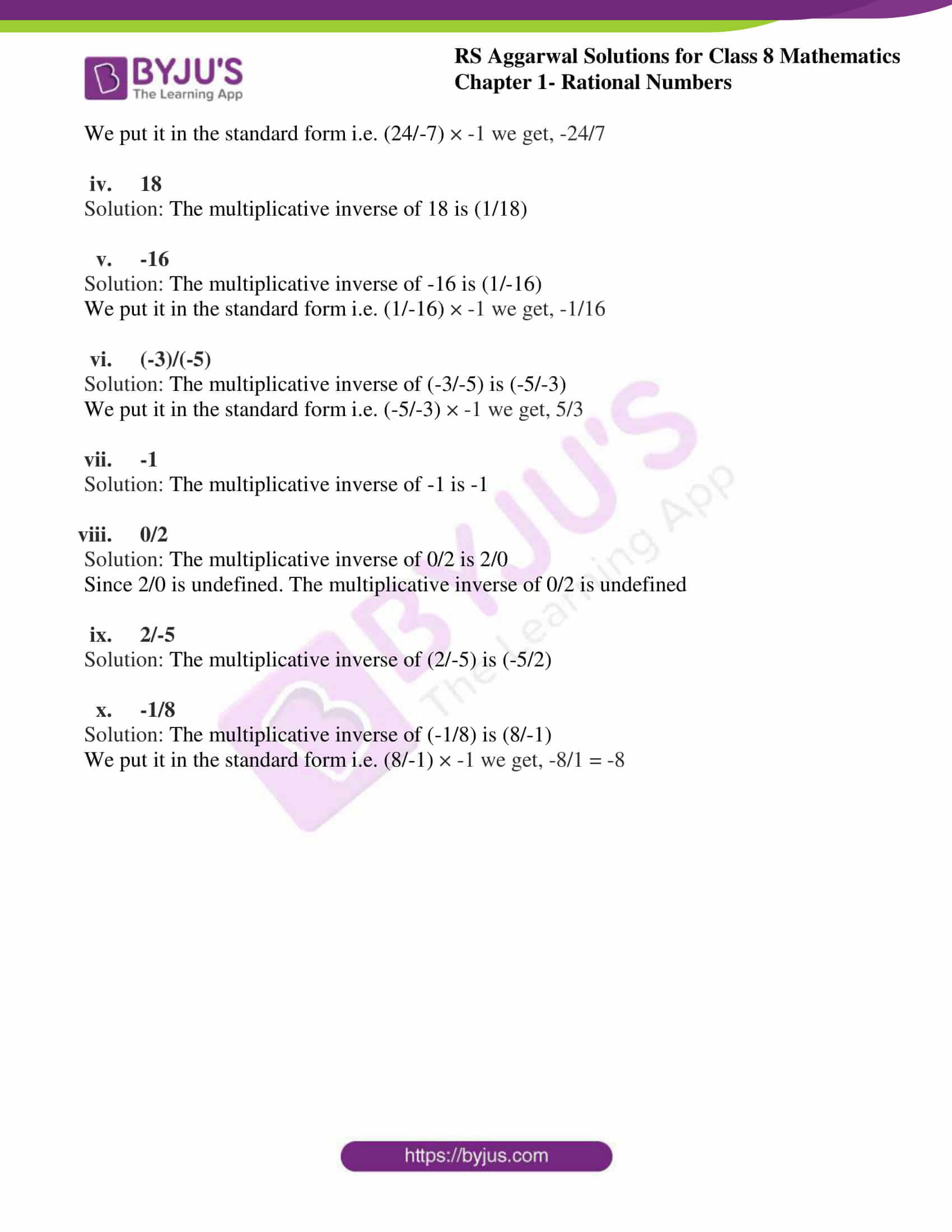### Access Answers to RS Aggarwal Solutions for Class 8 Maths Chapter 1- Rational Numbers Exercise 1D

Q1. Find each of the following products:

(i) (3/5) × (-7/8)

Solution:

(3/5) × (-7/8)

Here by multiplying numerators and denominators

We get

(3× -7) / (5 × 8) => -21/40

(ii) -9/2 × 5/4

Solution:

(-9/2) × (5/4)

Here by multiplying numerators and denominators

We get

(-9×5) / (2×4) => -45/8

(iii) (-6/11) × (-5/3)

Solution:

(-6/11) × (-5/3)

Here by multiplying numerators and denominators

We get

(-6×-5) / (11×3) = 30/33

further dividing to lowest terms by common divisor i.e. 3 = 30/33 => 10/11

(iv) (-2/3) × (6/7)

Solution:

(-2/3) × (6/7)

Here by multiplying numerators and denominators

We get

(-2×6) / (3×7) = -12/21

further dividing to lowest terms by common divisor i.e. 3 = -12/21 => -4/7

(v) (-12/5) × (10/-3)

Solution:

(-12/5) × (10/-3)

Here by multiplying numerators and denominators

We get (-12×10) / (5×-3) = -120/-15

further dividing to lowest terms by common divisor i.e. 3 = -120/-15 => 40/5

Further dividing to lowest terms by common divisor i.e. 5 = 40/5 => 8

(vi) (25/-9) × (3/-10)

Solution:

(25/-9) × (3/-10)

Here by multiplying numerators and denominators

We get

(25×3) / (-9×-10) = 75/90

Further dividing to lowest terms by common divisor i.e. 15 = 75/90 => 5/6

(vii) (5/-18) × (-9/20)

Solution:

(5/-18) × (-9/20)

Here by multiplying numerators and denominators

We get

(5×-9) / (-18×20) = -45/-360 = 45/360

Further dividing to lowest terms by common divisor i.e. 45 = 45/360 => 1/8

(viii) (-13/15) × (-25/26)

Solution:

(-13/15) × (-25/26)

Here by multiplying numerators and denominators

We get

(-13×-25) / (15×26) = 325/390

Further dividing to lowest terms by common divisor i.e. 5 = 325/390 = 65/78

Further dividing to lowest terms by common divisor i.e. 13 = 65/78 => 5/6

(ix) (16/-21) × (14/5)

Solution:

(16/-21) × (14/5)

Here by multiplying numerators and denominators

We get

(16×14) / (-21×5) = (224 ×-1) / (-105 ×-1) = -224/105

Further dividing to lowest terms by common divisor i.e. 7 = -224 /105 = -32/15

(x) (-7/6) × (24/1)

Solution:

Here by multiplying numerators and denominators

We get

(-7×24) / (6 ×1) = -168/6

Further dividing to lowest terms by common divisor i.e. 2 = -168/6 = -84/3

Further dividing to lowest terms by common divisor i.e. 3 = -84/3 => -28

(xi) (7/24) × (-48)

Solution:

Here by multiplying numerators and denominators

We get

(7×-48) / (24 ×1) = -336/24

Further dividing to lowest terms by common divisor i.e. 6 = -336/24 = -56/4

Further dividing to lowest terms by common divisor i.e. 6 = -84/6 => -14

(xii) (-13/5) / (-10)

Solution:

Here by multiplying numerators and denominators

We get

(-13×-10) / 5 = 130/5

Further dividing to lowest terms by common divisor i.e. 5 = 130/5 = 26

Q2.verify each of the following:

(i) (3/7) × (-5/9) = (-5/9) × (3/7)

Solution:

Firstly consider LHS

(3/7) × (-5/9) = (3×-5) / (7×9) = -15/63

Further by dividing -15/63 by using common divisor 3 we get, -5/21

RHS: (-5/9) × (3/7)

(-5/9) × (3/7) = (-5×3) / (9×7) = -15/63

Further by dividing -15/63 by using common divisor 3 we get, -5/21

∴ LHS = RHS is verified.

(ii) (-8/7) × (13/9) = (13/9) × (-8/7)

Solution:

Firstly consider LHS

(-8/7) × (13/9) = (-8×13) / (7×9) = -104/63

RHS: (13/9) × (-8/7)

(13/9) × (-8/7) = (13×-8) / (9×7) = -104/63

∴ LHS = RHS is verified.

(iii) (-12/5) × (7/-36) = (7/-36) × (-12/5)

Solution:

Firstly consider LHS

(-12/5) × (7/-36) = (-12×7) / (5×-36) = -84/-180 = 84/180

RHS: (7/-36) × (-12/5)

(7/-36) × (-12/5) = (7×-12) / (-36×5) = -84/-180 = 84/180

∴ LHS = RHS is verified.

(iv) -8 × (-13/12) = (-13/12) × -8

Solution:

Firstly consider LHS

-8 × (-13/12) = (-8×-13) / 12 = 104/12

Further by dividing 104/12 by using common divisor 4 we get, 26/3

RHS: (-13/12) × -8

(-13/12) × -8 = (-13×-8) / 12 = 104/12

Further by dividing 104/12 by using common divisor 4 we get, 26/3

∴ LHS = RHS is verified.

Q3.Verify each of the following:

(i) ((5/7) × (12/13)) × (7/18) = (5/7) × ((12/13) × (7/18))

Solution:

Firstly consider LHS

((5/7) × (12/13)) × (7/18)

((5×12) / (7×13)) × (7/18)

(60/91) × (7/18)

((60×7) / (91×18)) = 420/1638

Further by dividing 420/1638 by using common divisor 42 we get, 10/39

RHS: (5/7) × ((12/13) × (7/18))

(5/7) × ((12×7) / (13×18))

(5/7) × (84/234)

(5×84) / (7×234) = 420/1638

Further by dividing 420/1638 by using common divisor 42 we get, 10/39

∴ LHS = RHS is verified.

(ii) (-13/24) × ((-12/5) × (35/36)) = ((-13/24) ×(-12/5)) × (35/36)

Solution:

Firstly consider LHS

(-13/24) × ((-12/5) × (35/36))

(-13/24) × ((-12×35) / (5×36))

(-13/24) × (-420/180)

(-13×-420) / (24×180) = 5460/4320

RHS: ((-13/24) × (-12/5)) × (35/36)

((-13×-12) / (24×5)) × (35/36)

(156/120) × (35/36)

(156×35) / (120×36) = 5460/4320

Further by dividing 5460/4320 by using common divisor 10 we get, 546/432

Further by dividing 546/432 by using common divisor 6 we get, 91/72

∴ LHS = RHS is verified.

(iii) ((-9/5) × (-10/3)) × (21/-4) = (-9/5) × ((-10/3) × (21/-4))

Solution:

Firstly consider LHS

((-9/5) × (-10/3)) × (21/-4)

((-9×-10) / (5×3)) × (21/-4)

(90/15) × (21/-4)

((90×21) / (15×-4)) = (1890/-60) × -1 = -1890/60

Further by dividing -1890/60 by using common divisor 10 we get, -189/6

Further by dividing -189/6 by using common divisor 3 we get, -63/2

RHS: (-9/5) × ((-10/3) × (21/-4))

(-9/5) × ((-10×21) / (3×-4))

(-9/5) × (-210/-12)

(-9×-210) / (5×-12) = (1890/-60) × -1 = -1890/60

Further by dividing -1890/60 by using common divisor 10 we get, 189/6

Further by dividing -189/6 by using common divisor 3 we get, -63/2

∴ LHS = RHS is verified.

Q4. Fill in the blanks:

(i) (-23/17) × (18/35) = (18/35) × (….)

Solution:

we know the commutative law states that, a × b = b × a

By using the above law

(-23/17) × (18/35) = (18/35) × (-23/17)

(ii) -38 × (-7/19) = (-7/19) × (…)

Solution:

we know the commutative law states that, a × b = b × a

By using the above law

-38 × (-7/19) = (-7/19) × (-38)

(iii) ((15/7) × (-21/10)) × (-5/6) = (…) × ((-21/10) × (-5/6))

Solution:

we know the associative law states that, (a × b) × c = a × (b × c)

By using the above law

((15/7) × (-21/10)) × (-5/6) = (15/7) × ((-21/10) × (-5/6))

(iv) (-12/5) × ((4/15) × (25/-16)) = ((-12/5) × (4/5)) × (…)

Solution:

we know the associative law states that, (a × b) × c = a × (b × c)

By using the above law

(-12/5) × ((4/15) × (25/-16)) = ((-12/5) × (4/5)) × (25/-16)

Q5. Find the multiplicative inverse (i.e. reciprocal) of:

We know that the multiplicative inverse of a number (a/b) is (b/a)

(i) 13/25

Solution:

The multiplicative inverse of (13/25) is (25/13)

(ii) -17/12

Solution:

The multiplicative inverse of (-17/12) is (12/-17)

We put it in the standard form i.e. (12/-17) × -1 we get, -12/17

(iii) -7/24

Solution:

The multiplicative inverse of (-7/24) is (24/-7)

We put it in the standard form i.e. (24/-7) × -1 we get, -24/7

(iv) 18

Solution:

The multiplicative inverse of 18 is (1/18)

(v) -16

Solution:

The multiplicative inverse of -16 is (1/-16)

We put it in the standard form i.e. (1/-16) × -1 we get, -1/16

(vi) (-3)/(-5)

Solution:

The multiplicative inverse of (-3/-5) is (-5/-3)

We put it in the standard form i.e. (-5/-3) × -1 we get, 5/3

(vii) -1

Solution:

The multiplicative inverse of -1 is -1

(viii) 0/2

Solution:

The multiplicative inverse of 0/2 is 2/0

Since 2/0 is undefined.

The multiplicative inverse of 0/2 is undefined

(ix) 2/-5

Solution:

The multiplicative inverse of (2/-5) is (-5/2)

(x) -1/8

Solution:

The multiplicative inverse of (-1/8) is (8/-1)

We put it in the standard form i.e. (8/-1) × -1 we get, -8/1 = -8

### Access other Exercises of RS Aggarwal Solutions for Class 8 Maths Chapter 1- Rational Numbers

Exercise 1A Solutions 10 Questions

Exercise 1B Solutions 3 Questions

Exercise 1C Solutions 14 Questions

Exercise 1E Solutions 12 Questions

Exercise 1F Solutions 8 Questions

Exercise 1G Solutions 20 Questions

Exercise 1H Solutions 23 Questions

## RS Aggarwal Solutions for Class 8 Maths Chapter 1- Rational Numbers Exercise 1D

Exercise 1D of RS Aggarwal Class 8 Chapter 1, Rational Numbers contains the advance concepts related to Rational Numbers. This exercise mainly deals with the Multiplication of rational numbers, use of value 0 when multiplied in the fractions, the Distribution of Rational numbers and reciprocal of the fractions.

Practicing The RS Aggarwal Solutions textbook can help the students in a better understanding of the concepts and helps in securing high marks in your examination.